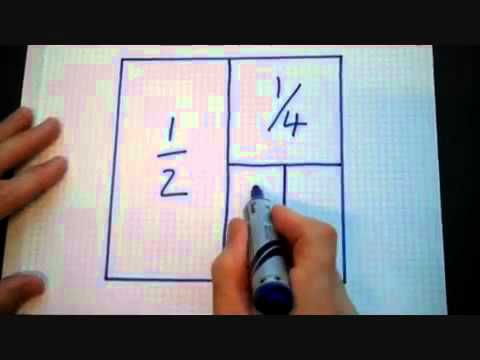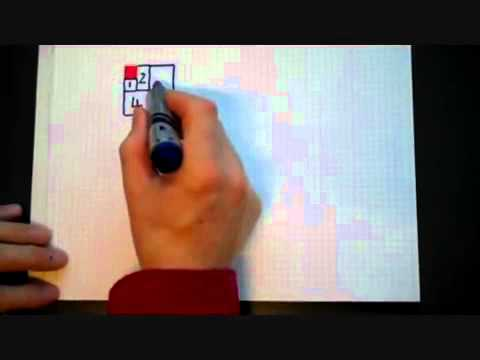### Submit a Resource

NRICH: Double TroubleCharlie has been adding fractions in the sequence 12,14,18, where each fraction is half the previous one:

1/2+1/4
1/2+1/4+1/8
12+14+18+116

Work out the answers to Charlie’s sums. What do you notice?

Will the pattern continue?
How do you know?

Try writing an expression for

1/2+1/4+1/8++1/2n

Could you convince someone else that your expression is correct for all values of n?

Charlie drew a diagram to try to explain what was going on (video).

Use Charlie’s diagram to explain why

1/2+1/4+1/8++1/2n=11/2n=2n1/2n

Alison has been adding numbers in the sequence 1,2,4,8, where each number is twice the previous one:

1+2
1+2+4
1+2+4+8

Work out the answers to Alison’s sums. What do you notice?

Will the pattern continue?

How do you know?

Try writing an expression for

1+2+4++2n

Could you convince someone else that your expression is correct for all values of n?

Alison drew a diagram to try to explain what was going on (see video).

Can you use Alison’s diagram to explain why

1+2+4++2n=2n+11

Age 14 to 16

Algebra & Pre-Algebra, Exponential Equations, Patterning & Sequencing
High School, Educator

## Organization

NRICH (University of Cambridge)

Challenge